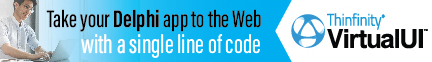DelphiBasicsDecProcedure Decrement an ordinal variable System unit
 1 procedure Dec ( var Variable : Ordinal variable ) ; 2 procedure Dec ( var Variable : Ordinal variable; Count : Integer ) ;
Description
The Dec procedure decrements the ordinal Variable parameter passed to it.

You can decrement :

 Characters Non-floating number types Enumeration types Pointers

The decrement is by the base size of the unit. For example, decrementing a Pointer will be by 4 bytes if the pointer points to Words.

Version 1 of Inc decrements by 1 unit.

Version 2 of Inc decrements by Count units.

Notes
Dec is equivalent in performance to simple subtraction, or the Pred procedure.

Count can be negative.
Related commands
 Inc Increment an ordinal variable Pred Decrement an ordinal variable Sqr Gives the square of a number Sqrt Gives the square root of a number Succ Increment an ordinal variable Succ Increment an ordinal variable Sum Return the sum of an array of floating point valuesDownload this web site as a Windows program.